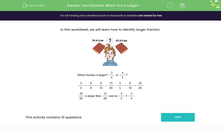# Identify the Larger Fraction

In this worksheet, students will decide which of two fractions is the larger.Key stage:  KS 2

Curriculum topic:   Number: Fractions, Decimals and Percentages

Curriculum subtopic:   Compare and Order Fractions

Difficulty level:#### Worksheet Overview

In this activity, we will be learning how to identify the larger fraction.Which fraction is larger 3/4 or 4/5?

We can't compare these fractions at the moment because they have different denominators - it would be like comparing 3 apples and 4 oranges!

So, the first thing we have to do is to make the denominators the same - in other words, find a common denominator.

What's the lowest common multiple of 4 and 5? It's 20, so we'll make that our new denominator.

Now we need to look at what we did to each denominator to turn it into 20 and do the same thing to the numerator.

With 3/4, we multiplied the 4 by 5 to make 20, so we need to multiply the 3 by 5 too. This gives us 15/20.

With 4/5, we multiplied the 5 by 4 to make 20, so we need to multiply the 4 by 4 too. This gives us 16/20.

We now have 15/20 and 16/20. It is clear that 16/20 is larger than 15/20, so this means that 4/5 is larger than 3/4.

Now that we have a common denominator, we can do all sorts of things with these fractions, not just comparing, but also adding and subtracting. So, all in all, a very useful skill to have!

Let's practise it in the following questions!### What is EdPlace?

We're your National Curriculum aligned online education content provider helping each child succeed in English, maths and science from year 1 to GCSE. With an EdPlace account you’ll be able to track and measure progress, helping each child achieve their best. We build confidence and attainment by personalising each child’s learning at a level that suits them.

Get started